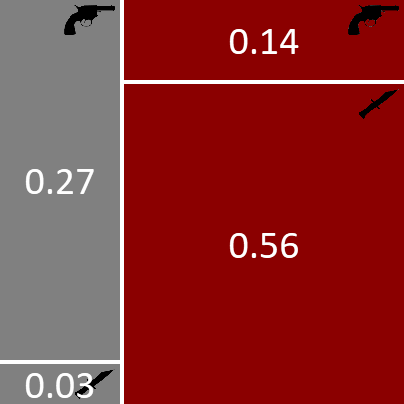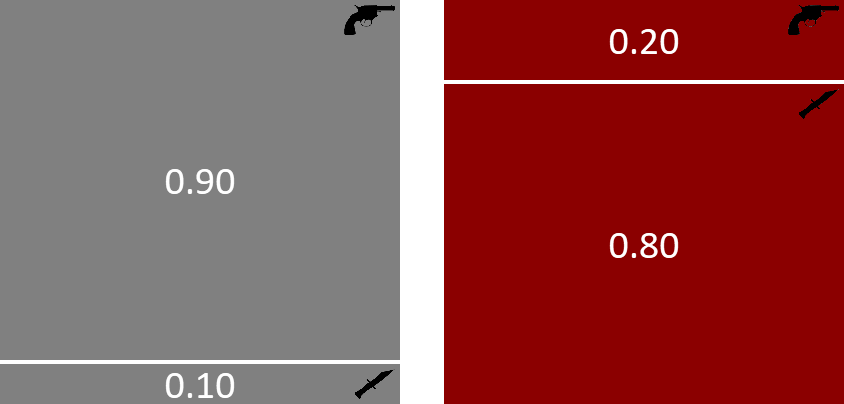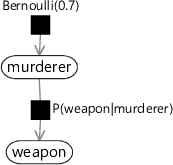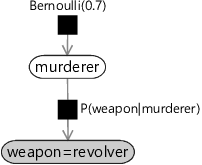## 1.3 Working with larger models

We now wish to incorporate more evidence from the crime scene – for each new piece of evidence that we consider, we will need to introduce another random variable into our model. So far we’ve only had to cope with a model of just two random variables: murderer and weapon. For this two-variable model, we were able to write the joint distribution pictorially, like so:=$\times$$P(\var{weapon}, \var{murderer})$   $P(\var{murderer})$   $P(\var{weapon} | \var{murderer})$
Figure 1.8The joint distribution for our two-variable model, shown as a product of two factors.

Unfortunately, if we increase the number of random variables in our model beyond two (or maybe three), we cannot represent the joint distribution using this pictorial notation. But in real models there will typically be anywhere from hundreds to hundreds of millions of random variables. We need a different notation to represent and work with such large joint distributions.

The notation that we will use exploits the fact that most joint distributions can be written as a product of a number of terms or factors each of which refers to only a small number of variables. For example, our joint distribution above is the product of two factors: $P(\var{murderer})$ which refers to one variable and $P(\var{weapon} | \var{murderer})$ which refers to two variables. Even for joint distributions with millions of variables, the factors which make up the distribution usually refer to only a few of these variables. As a result, we can represent a complex joint distribution using a factor graph that shows which factors make up the distribution and what variables those factors refer to.

Figure 1.9 shows a factor graph for the two-variable joint distribution above. There are two types of node in the graph: a variable node for each variable in the model and a factor node for each factor in the joint distribution. Variable nodes are shown as white ellipses (or rounded boxes) containing the name of the variable. Factor nodes are small black squares, labelled with the factor that they represent. We connect each factor node to the variable nodes that it refers to. For example, the $P(\var{murderer})$ factor node is connected only to the $\var{murderer}$ variable node since that is the only variable it refers to, whilst $P(\var{weapon} | \var{murderer})$ connects to both the weapon and murderer variable nodes, since it refers to both variables. Finally, if the factor defines a distribution over one of its variables, we draw an arrow on the edge pointing to that variable (the child variable). If the factor defines a conditional distribution, the other edges from that factor connect to the variables being conditioned on (the parent variables) and do not have arrows.

murdererweaponP(murderer)P(weapon|murderer)Figure 1.9Factor graph for the murder mystery model. The model contains two random variables murderer and weapon, shown as white nodes, and two factors $P(\var{murderer})$ and $P(\var{weapon} | \var{murderer})$, shown as black squares.

The factor graph of Figure 1.9 provides a complete description of our joint probability, since it can be found by computing the product of the distributions represented by the factor nodes. As we look at more complex factor graphs throughout the book, it will always hold that the joint probability distribution over the random variables (represented by the variable nodes) can be written as the product of the factors (represented by the factor nodes). The joint distribution gives a complete specification of a model, because it defines the probability for every possible combination of values for all of the random variables in the model. Notice that in Figure 1.8 the joint distribution was represented explicitly, but in the factor graph it is represented only indirectly, via the factors.

Since we want our factor graphs to tell us as much as possible about the joint distribution, we should label the factors as precisely as possible. For example, since we know that $P(\var{murderer})$ defines a prior distribution of $\dist{Bernoulli}(0.7)$ over murderer, we can label the factor “Bernoulli(0.7)”. We do not need to mention the murderer variable in the factor label since the factor is only connected to the murderer variable node, and so the distribution must be over murderer. This allows more informative labelling of the factor graph, like so:

murdererweaponBernoulli(0.7)P(weapon|murderer)Figure 1.10Factor graph representation of the murder mystery model with the Bernoulli prior over murderer labelled explicitly.

In this book, we will aim to label factors in our factor graphs so that the function represented by each factor is as clear as possible.

There is one final aspect of factor graph notation that we need to cover. When doing inference in our two-variable model, we observed the random variable weapon to have the value revolver. This step of observing random variables is such an important one in model-based machine learning that we introduce a special graphical notation to depict it. When a random variable is observed, the corresponding node in the factor graph is shaded (and sometimes also labelled with the observed value), as shown for our murder mystery in Figure 1.11.

weapon=revolvermurdererBernoulli(0.7)P(weapon|murderer)Figure 1.11The factor graph for the murder mystery, with the weapon node shaded to indicate that this random variable has been observed, and is fixed to the value revolver.

Representing a probabilistic model using a factor graph gives many benefits:

• It provides a simple way to visualize the structure of a probabilistic model and see which variables influence each other.
• It can be used to motivate and design new models, by making appropriate modifications to the graph.
• The assumptions encoded in the model can be clearly seen and communicated to others.
• Insights into the properties of a model can be obtained by operations performed on the graph.
• Computations on the model (such as inference) can be performed by efficient algorithms that exploit the factor graph structure.

We shall illustrate these points in the context of specific examples throughout this book.

### Inference without computing the joint distribution

Having observed the value of weapon, we previously computed the full joint distribution and used it to evaluate the posterior distribution of murderer. However, for most real-world models it is not possible to do this, since the joint distribution would be over too many variables to allow it to be computed directly. Instead, now that we have our joint distribution represented as a product of factors, we can arrive at the same result by using only the individual factors – in a way which is typically far more efficient to compute. The key lies in applying the product and sum rules of probability in an appropriate way. From the product rule (1.15) we have

However, by symmetry we can equally well write

Equating the right-hand sides of these two equations and re-arranging we obtain

This is an example of Bayes' theorem or Bayes’ rule [Bayes, 1763] which plays a fundamental role in many inference calculations (see Panel 1.1). Here $P(\var{murderer})$ is the prior probability distribution over the random variable murderer and is one of the things we specified when we defined our model for the murder mystery. Similarly, $P(\var{weapon} | \var{murderer})$ is also something we specified, and is called the likelihood function and should be viewed as a function of the random variable murderer. The quantity on the left-hand side $P(\var{murderer} | \var{weapon})$ is the posterior probability distribution over the murderer random variable, i.e. the distribution after we have observed the evidence of the revolver.

The denominator $P(\var{weapon})$ in equation (1.24) plays the role of a normalization constant and ensures that the left hand side of Bayes’ theorem is correctly normalized (i.e. adds up to 1 when summed over all possible states of the random variable murderer). It can be computed from the prior and the likelihood using

which follows from the product and sum rules. When working with Bayes’ rule, it is sometimes useful to drop this denominator $P(\var{weapon})$ and instead write

where $\propto$ means that the left-hand side is proportional to the right-hand side (i.e. they are equal up to a constant that does not depend on the value of murderer). We do not need to compute the denominator because the normalization constraint tells us that the conditional probability distribution $P(\var{murderer} | \var{weapon})$ must add up to one across all values of murderer. Once we have evaluated the right hand side of (1.26) to give a number for each of the two values of murderer, we can scale these two numbers so that they sum up to one, to get the resulting posterior distribution.

Now let us apply Bayes’ rule to the murder mystery problem. We know that weapon=revolver, so we can evaluate the right hand side of equation (1.26) for both murderer=Grey and murderer=Auburn giving:

These numbers sum to 0.41. To get probabilities, we need to scale both numbers to sum to 1 (by dividing by 0.41) which gives:

This is the same result as before. Although we have arrived at the same result by a different route, this latter approach using Bayes’ theorem is preferable as we did not need to compute the joint distribution. With only two random variables so far in our murder mystery this might not look like a significant improvement, but as we go to more complex problems we will see that successive applications of the rules of probability allows us to work with small sub-sets of random variables – even in models with millions of variables!

Self assessment 1.3

The following exercises will help embed the concepts you have learned in this section. It may help to refer back to the text or to the concept summary below.

1. Use Bayes’ theorem to compute the posterior probability over murderer, for the case that the murder weapon was the dagger rather than the revolver. Compare this to your answer from the previous self assessment.
2. For the scenario you chose in the previous self assessment, draw the factor graph corresponding to the joint distribution. Ensure that you label the factors as precisely as possible. Verify that the product of factors in the factor graph is equal to the joint distribution.
3. Repeat the inference task from the previous self assessment (computing the posterior probability of the conditioning variable) using Bayes’ theorem rather than using the joint distribution. Check that you get the same answer as before.

factorsFunctions (usually of a small number of variables) which are multiplied together to give a joint probability distribution (which may be over a large number of variables). Factors are represented as small black squares in a factor graph.

factor graphA representation of a probabilistic model which uses a graph with factor nodes (black squares) for each factor in the joint distribution and variable nodes (white, rounded) for each variable in the model. Edges connect each factor node to the variable nodes that it refers to.

For example, here is a factor graph from Chapter 1:

murdererweaponP(murderer)P(weapon|murderer)variable nodeA node in a factor graph that represents a random variable in the model, shown as a white ellipse or rounded box containing the variable name.

factor nodeA node in a factor graph that represents a factor in the joint distribution of a model, shown as a small black square labelled with the factor name.

child variableFor a factor node, the connected variable that the arrow points to. This indicates that the factor defines a probability distribution over this variable, possibly conditioned on the other variables connected to this factor. The child variable for a factor is usually drawn directly below the factor.

parent variablesFor a factor node, the connected variable(s) with edges that do not have arrows pointing to them. When a factor defines a conditional probability distribution, these are the variables that are conditioned on. The parent variables for a factor are usually drawn above the factor.

Bayes' theoremThe fundamental theorem that lets us do efficient inference in probabilistic models. It defines how to update our belief about a random variable A after receiving new information B, so that we move from our prior belief $P(\var{A})$ to our posterior belief given B, $P(\var{A}|\var{B})$.

See Panel 1.1 for more details.

likelihood functionA conditional probability viewed as a function of its conditioned variable. For example, $P(\var{B}|\var{A})$ can be viewed as a function of A when B is observed and we are interested in inferring A. It is important to note that this not a distribution over A, since $P(\var{B}|\var{A})$ does not have to sum to 1 over all values of A. To get a distribution over A from a likelihood function, you need to apply Bayes’ theorem (see Panel 1.1).

References

[Bayes, 1763] Bayes, T. (1763). An Essay towards Solving a Problem in the Doctrine of Chances. By the Late Rev. Mr. Bayes, F. R. S. Communicated by Mr. Price, in a Letter to John Canton, A. M. F. R. S. Philosophical Transactions, 53:370–418.